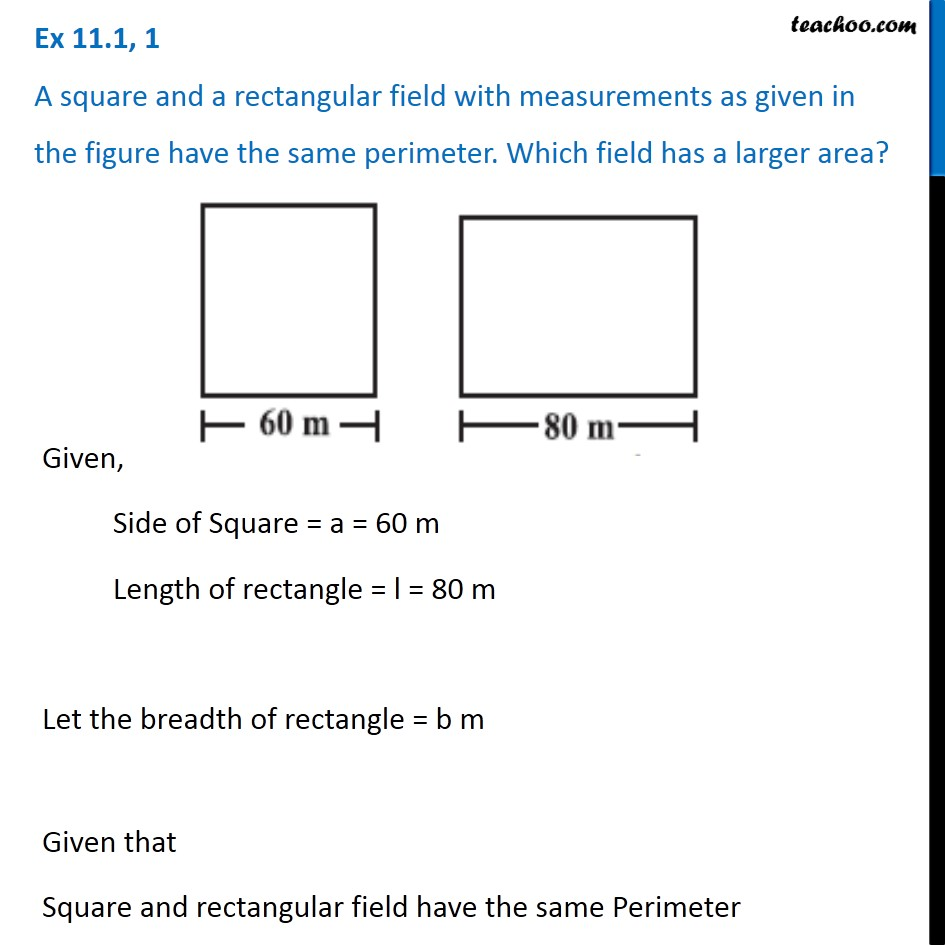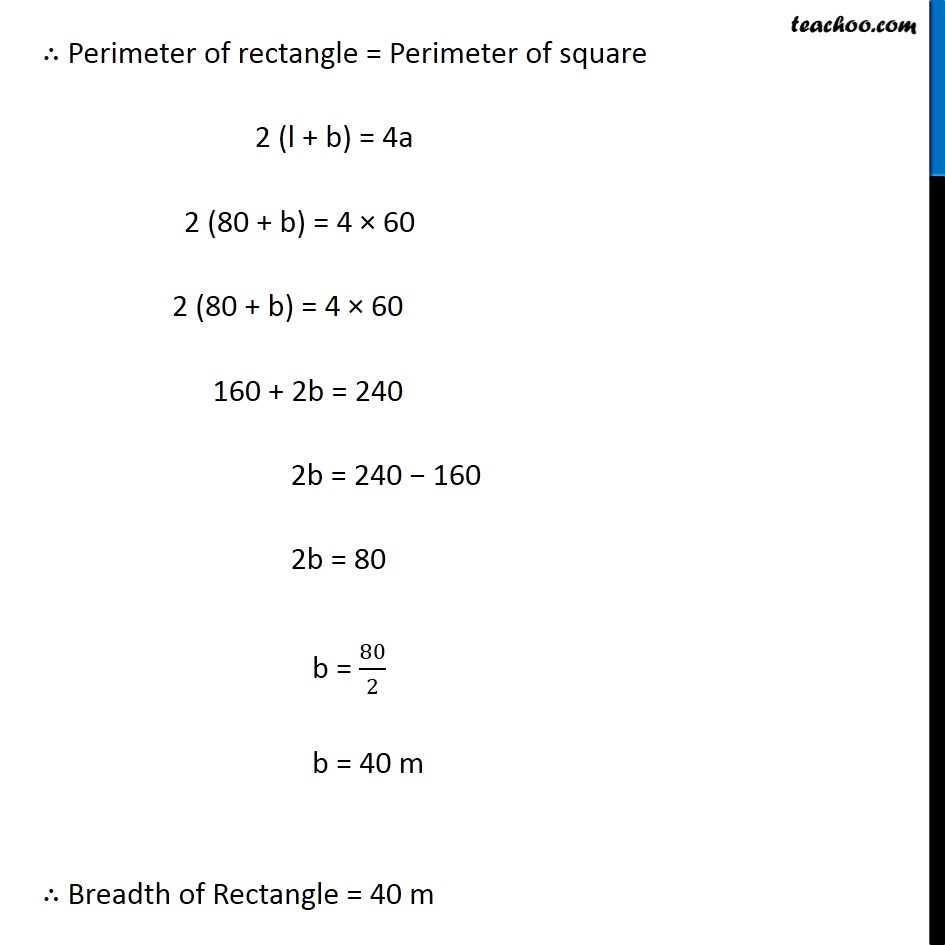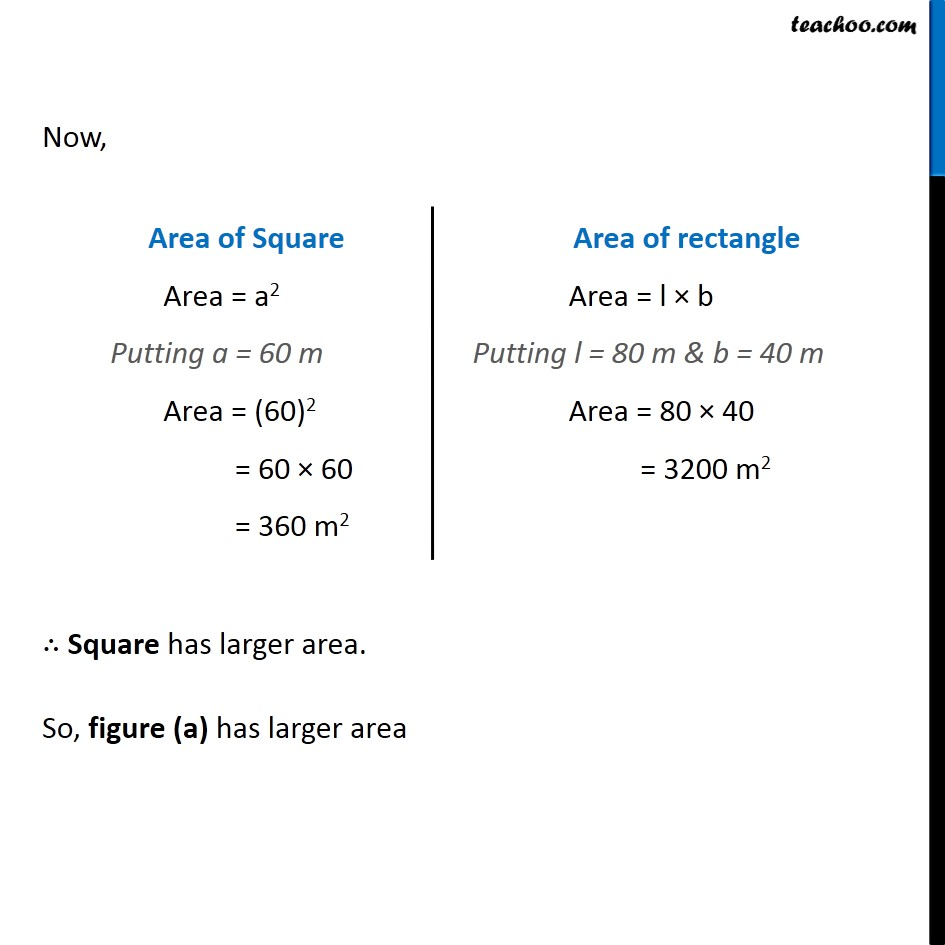Area and Perimeter

Chapter 9 Class 8 Mensuration
Concept wiseLearn in your speed, with individual attention - Teachoo Maths 1-on-1 Class

### Transcript

Question 1 A square and a rectangular field with measurements as given in the figure have the same perimeter. Which field has a larger area? Given, Side of Square = a = 60 m Length of rectangle = l = 80 m Let the breadth of rectangle = b m Given that Square and rectangular field have the same Perimeter ∴ Perimeter of rectangle = Perimeter of square 2 (l + b) = 4a 2 (80 + b) = 4 × 60 2 (80 + b) = 4 × 60 160 + 2b = 240 2b = 240 − 160 2b = 80 b = 80/2 b = 40 m ∴ Breadth of Rectangle = 40 m Now, Area of Square Area = a2 Putting a = 60 m Area = (60)2 = 60 × 60 = 360 m2 Area of rectangle Area = l × b Putting l = 80 m & b = 40 m Area = 80 × 40 = 3200 m2 ∴ Square has larger area. So, figure (a) has larger area